# 3 and 4 Marks Question

1. Draw a ray diagram of an image formed when an object is placed at a distance of 10 cm from a convex lens having a focal length of 20 cm. Write any two nature of the image. Calculate the power of the lens.
2. A ray diagram of an image formed when an object is placed at a distance of 10 cm from a convex lens having focal length 20 cm is shown below: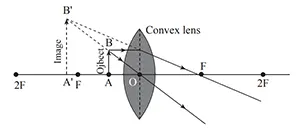Nature of image is virtual, erect and magnified.

Here,
focal length (f) = 20 cm = 0.2 m
power (p) = ?

Now, we have,
p = $\frac{1}{f}$
p = $\frac{1}{0.2}$
∴ p = 5 D

3. Complete the ray diagram given alongside. Also mention any two natures of the image thus formed. If the power of the lens is +4D, what should be the distance between lens and magazine to read it properly?
4.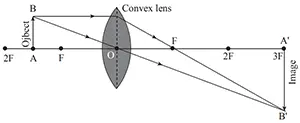Its nature is real, inverted and magnified.

Here,
Power (p) = +4D
distance between lens and magazine = focal length (f) = ?
Now, we know,
f = $\frac{1}{p}$
f = $\frac{1}{4}$
∴ f = 0.25 m = 25 cm
5. Draw a ray diagram of an image formed when an object kept at a distance of 50 cm from a convex lens having focal length 20 cm. Write any two nature of image. Calculate the power of lens.
6. A ray diagram of an image formed when an object kept at a distance of 50 cm from a convex lens having focal length 20 cm is shown below: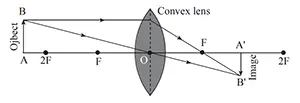Nature of image formed is real, inverted and diminished.

Here,
focal length (f) = 20 cm = 0.2 m
power (p) = ?
Now, we have,
f = $\frac{1}{p}$
p = $\frac{1}{0.2}$
∴ p = 5D
7. Study the given diagram and answer the following questions.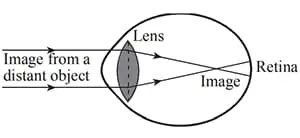1. What type of defect of vision is in the diagram of eye?
2. The type of defect of vision is in the diagram of eye is short sightedness.
3. What is the cause of this defect of vision?
4. The cause of this defect of vision is listed below:
1. Due to the decrease in focal length of the eye lens.
2. Due to the elongation of eye ball.
5. What is to be done to remedy such defect?
6. We can remedy such defect by wearing spectacles with concave lens of suitable power.
7. Draw a diagram to show the remedy of this defect.
8. A diagram to show the remedy of this defect is shown below: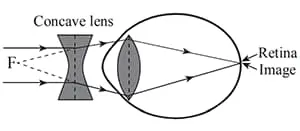8. Draw a ray diagram of an image formed when an object kept at a distance of 40 cm from a convex lens having focal length 20 cm. Write any two nature of image. Calculate the magnification of image.
9. A ray diagram of an image formed when an object kept at a distance of 40 cm from a convex lens having focal length 20 cm is: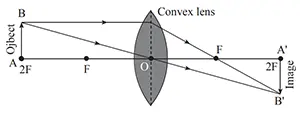Nature of image formed is real, inverted and same size as that of the object.

Here,
As size of the object and image is same,
∴ Magnification =

$\frac{\text{height of image}}{\text{height of object}}$ = 1 = $\frac{\text{image distance}}{\text{object distance}}$

10. Draw a ray diagram of an image formed when an object is placed at a distance of 20 cm from a convex lens having focal length 20 cm. Write any two natures of image formed. Calculate the magnification of image.
11. A ray diagram of an image formed when an object is placed at a distance of 20 cm from a convex lens having focal length 20 cm is: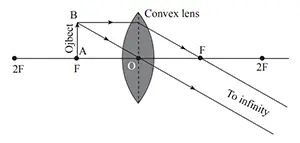Nature of image formed is real, inverted and highly magnified.

Here,
height of object (O) = 20 cm
height of image (I) ∞
magnification (m) = ?

Now,
m = $\frac{I}{O}$
∴ m = ∞

12. Calculate the ray diagram of image formed by convex lens having focal length 2 cm, if lighting candle is placed 4 cm away from lens. Also calculate the magnification of lens.
13. The ray diagram of image formed by convex lens having focal length 2 cm, if lighting candle is placed 4 cm away from lens is:Here,
Image distance (v) = 4 cm
Object distance (u) = 4 cm
Magnification (m) = ?
Now, we have,

m = $\frac{\text{v}}{\text{u}}$
= $\frac{4}{4}$
= 1
∴ m = 1

14. Power of the given lens is +25D. Answer the following questions based on it.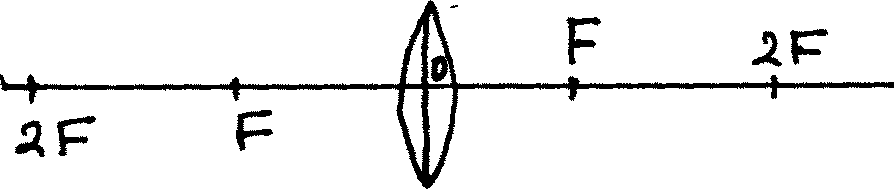1. Find the distance FO.
2. Here,
Power (p) = +25 D
distance FO = focal length (f) = ?
We know,
f = $\frac{1}{\text{p}}$
f = $\frac{1}{25}$
∴ FO = f = 0.04 m = 4 cm
3. Draw a ray diagram of the image formed by this lens when an object is kept 7 cm away from the lens. Also write any three natures of the image so formed.
4. A ray diagram of the image formed by this lens when an object is kept 7 cm away from the lens is:Nature of image formed is real, inverted and magnified.
15. Observe the defect of vision in the given diagram and answer the following questions.1. What is the cause of this defect?
2. The cause of this defect (short sightedness) are listed below:
1. Due to the decrease in focal length of the eye lens.
2. Due to the elongation of eye ball.
3. How can this defect be corrected? Illustrate with a ray diagram.
4. This defect can be corrected by wearing spectacles with concave lens of suitable power.